DigDB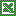Add-in Tools for Excel Elites
Who else wants to do a 10-hour Excel analysis in 15 minutes? (more ...)

Features | Testimonial | Download free 15-day trial | Purchase | FAQ | Contact

### Rounding values in a range or column in Excel - Round, RoundUp, RoundDown, Floor, Ceiling, Int, Even, Odd

Excel offers a variety of rounding formulas that allow a user to round a number to just about any approximation. Below is a list and rounding options. But the problem is that you cannot easily apply these formulas to an arbitrary range or table column. DigDB will allow you to do this:
• You can select a range, then invoke 'DigDB->Selected Area->Rounding...', the cells in the selected range will be rounded in-place.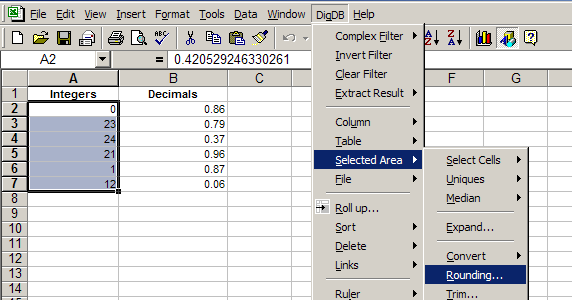Practice file - rounding-demo.xls (16k)

• Or, you can select a single cell in a table column, then invoke 'DigDB->Column->Rounding...', the entire column will be rounded, and the rounded values will show in a new column inserted next to the original column.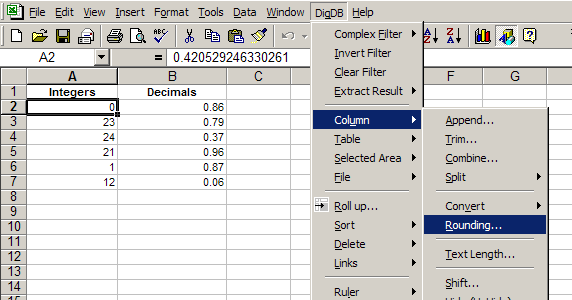Rounding is especially useful when you want a fuzzy match between two tables. For example, if one table's column has a value '2.4', and the other table's column has a value '2.2', and you only care if the two values match in the integer part, then you can round up the 2 columns first, then do the match.

### Rounding options

In the rounding command window, use the dropdown box to select how to round. You have the following rounding options: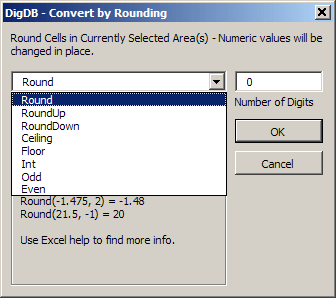Examples of Rounding results are shown when you select how to round.

### Round - rounds a number to a specified number of digits.

• If number of digits is > 0 (zero), then number is rounded to the specified number of decimal places.
• If number of digits is = 0, then number is rounded to the nearest integer.
• If number of digits is < 0, then number is rounded to the left of the decimal point. For example, Rounding 21.5 by -1 digits equals to rounding the number to the nearest 10s, that is 20.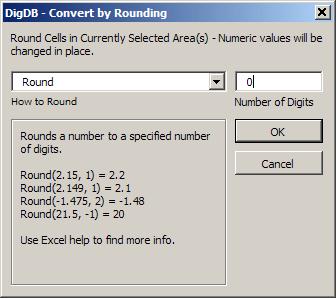### RoundUp - rounds a number up, away from 0 (zero).

ROUNDUP behaves like ROUND, except that it always rounds a number up.
• If number of digits is > 0 (zero), then number is rounded up to the specified number of decimal places.
• If number of digits is = 0, then number is rounded up to the nearest integer.
• If number of digits is < 0, then number is rounded up to the left of the decimal point. For example, Rounding 31415.92654 by -2 digits equals 31,500.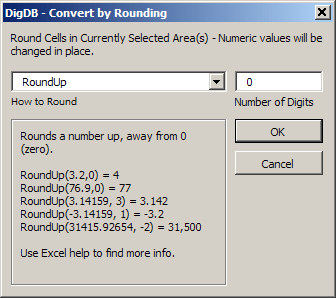### RoundDown - rounds a number down, toward 0 (zero)

ROUNDUP behaves like ROUND, except that it always rounds a number down.
• If number of digits is > 0 (zero), then number is rounded down to the specified number of decimal places.
• If number of digits is = 0, then number is rounded down to the nearest integer.
• If number of digits is < 0, then number is rounded down to the left of the decimal point. For example, Rounding 31415.92654 by -2 digits equals 31,400.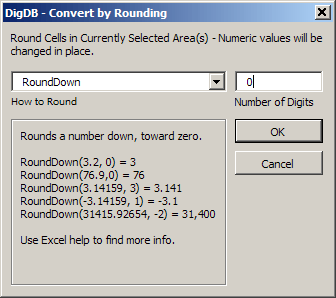### Ceiling

Returns number rounded up, away from zero, to the nearest multiple of significance. For example, if you want to avoid using pennies in your prices and your product is priced at \$4.42, CEILING 4.42 with significance of 0.05 will round prices up to the nearest nickel.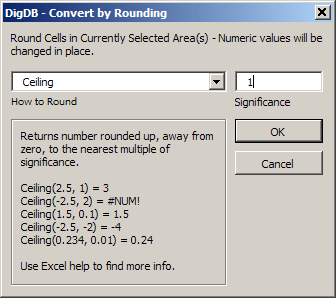### Floor

Rounds number down, toward zero, to the nearest multiple of significance.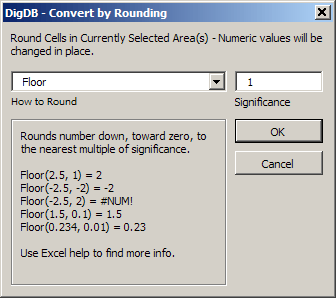### Int

Rounds a number down to the nearest integer.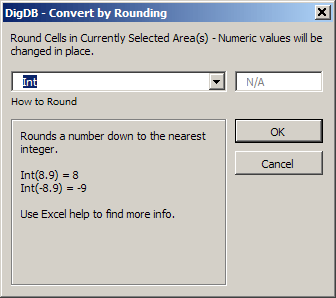### Odd

Returns number rounded up to the nearest odd integer.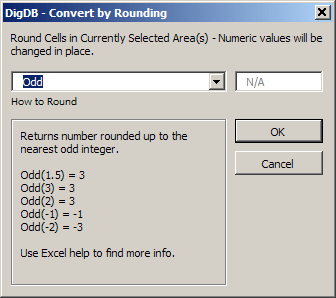### Even

Returns number rounded up to the nearest even integer.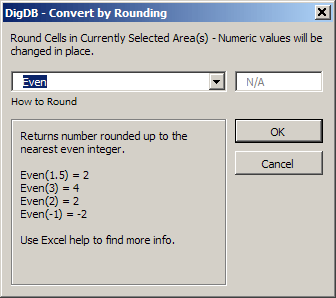### Need more?

• Browse the complete list of DigDB's powerful features listed on the front page.
• If you see errors or things not working, check out our error reference page.

DigDBAdd-in Tools for Excel Elites